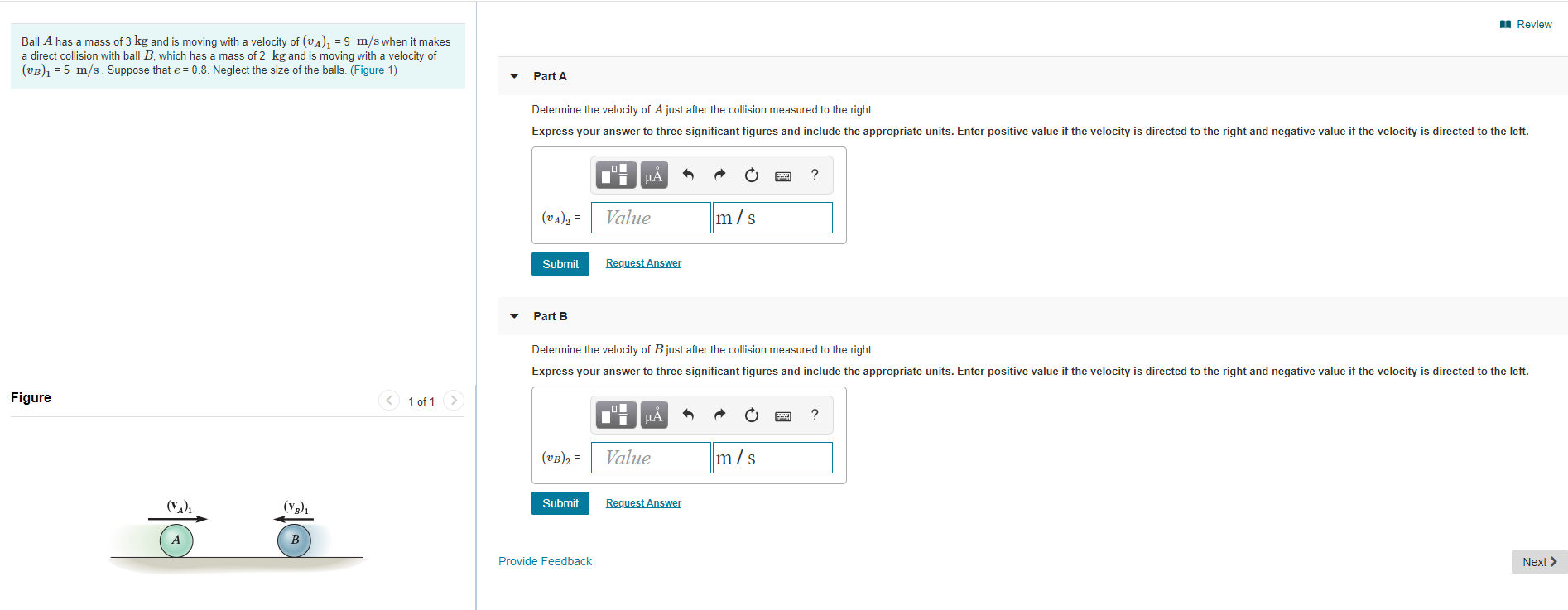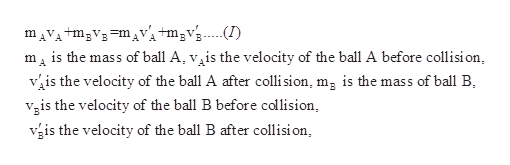# ReviewBall A has a mass of 3 kg and is moving with a velocity of (vA), =9 m/s when it makesa direct collision with ball B, which has a mass of 2 kg and is moving with a velocity of(vB5 m/s Suppose that e 0.8. Neglect the size of the balls. (Figure 1)Part ADetermine the velocity of Ajust after the collision measured to the right.Express your answer to three significant figures and include the appropriate units. Enter positive value if the velocity is directed to the right and negative value if the velocity is directed to the left.?m/sValue(UA)2=Request AnswerSubmitPart BDetermine the velocity of Bjust after the collision measured to the right.Express your answer to three significant figures and include the appropriate units. Enter positive value if the velocity is directed to the right and negative value if the velocity is directed to the left.Figure1 of 1m/sValue(VB)2(у)SubmitRequest AnswerBProvide FeedbackNext

Questionhelp_outlineImage TranscriptioncloseReview Ball A has a mass of 3 kg and is moving with a velocity of (vA), =9 m/s when it makes a direct collision with ball B, which has a mass of 2 kg and is moving with a velocity of (vB5 m/s Suppose that e 0.8. Neglect the size of the balls. (Figure 1) Part A Determine the velocity of Ajust after the collision measured to the right. Express your answer to three significant figures and include the appropriate units. Enter positive value if the velocity is directed to the right and negative value if the velocity is directed to the left. ? m/s Value (UA)2= Request Answer Submit Part B Determine the velocity of Bjust after the collision measured to the right. Express your answer to three significant figures and include the appropriate units. Enter positive value if the velocity is directed to the right and negative value if the velocity is directed to the left. Figure 1 of 1 m/s Value (VB)2 (у) Submit Request Answer B Provide Feedback Next fullscreen
check_circleExpert Solution
Step 1

(a)

Apply the law of conservation of momentumhelp_outlineImage TranscriptionclosemAVAtm3V3mVtm2vs...I) m is the mass of ball A, v^is the velocity of the ball A before collision is the velocity of the ball A after colli sion, mg is the mass of ball B is the velocity of the ball B before collision, is the velocity of the ball B after collisi on fullscreen
Step 2

The coefficient of restitution is given as

Step 3

Rewrite equation (II) after s...

### Want to see the full answer?

See Solution

#### Want to see this answer and more?

Solutions are written by subject experts who are available 24/7. Questions are typically answered within 1 hour*

See Solution
*Response times may vary by subject and question
Tagged in

### Collisions